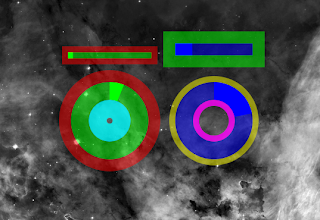## Sunday, February 14, 2010

This approach has the advantage of being much more compact and using less functions. I will be feeding the variables straight into the drawing function. The lua is to create bars and circle charts like so.You can get the whole code here on the crunchbang forum.

Here is the code lines in question:
The first line of the function that draws the bars is:
function draw_bar(co, width, height, across, down, bgr, bgg, bgb, bga, inr, ing, inb, ina, lw, lr, lg, lb, la, rotate)
you can see the name of the function followed by a long list of strings. These are all the strings that will be set later when the function is called. Here is a description of each string:

co = conky object data to display
width = how wide the rectangle will be
height = how tall the rectangle will be
across = x position
down = y position
bgr = red component of background color
bgg =green component of background color
bgb = blue component of background color
bga = alpha component of background color
inr = red component of indicator color
ing = green component of indicator color
inb = blue component of indicator color
ina = alpha component of indicator color
lw = width of the boundary line
lr = red component of line color
lg = green component of line color
lb = blue component of line color
la = alpha component of line color
rotate = rotation in degrees that you want applied to the bar

pretty straightforward variables, and I tried to make it so that you could more or less work out what the string names meant. Now here is the line when the function is called:
draw_bar(cpu, 150, 20, 75, 50, 0, 1, 0, 0.5, 0, 1, 0, 1, 10, 1, 0, 0, 0.5, 0)
Each value is passed up to the draw_bar function.
So that:
co=cpu
width=150
length=20
across=75
down=50
...etc etc.

Then you can call the same function again but feed it a different set of information like so:
draw_bar(mem, 150, 40, 250, 30, 0, 0, 1, 0.5, 0, 0, 1, 1, 20, 0, 1, 0, 0.5, 0)

Not as user friendly as the settings table approach but far less code and alot less complicated. Another important aspect to this approach is that it is more like a "tool" that can be used in a Lua script that contains multiple elements. All you need is the figure drawing function above the function that you will be calling in conky, then if you want a bar or circle meter in your setup, call the function with a single line setup.